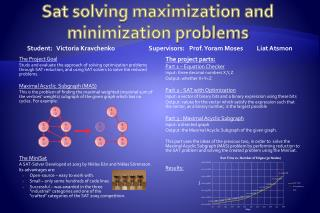Download PresentationSat solving maximization and minimization problems

# Sat solving maximization and minimization problems

Download Presentation## Sat solving maximization and minimization problems

- - - - - - - - - - - - - - - - - - - - - - - - - - - E N D - - - - - - - - - - - - - - - - - - - - - - - - - - -
##### Presentation Transcript

1. Sat solving maximization and minimization problems • Student: Victoria Kravchenko Supervisors: Prof. Yoram Moses LiatAtsmon The Project Goal Study and evaluate the approach of solving optimization problems through SAT reduction, and using SAT solvers to solve the reduced problems. Maximal Acyclic Subgraph (MAS) This is the problem of finding the maximal weighted (maximal sum of the vertices’ weights) subgraph of the given graph which has no cycles. For example: The MiniSat A SAT-Solver Developed at 2003 by NiklasEén and NiklasSörensson. Its advantages are: • Open-source – easy to work with. • Small – only some hundreds of code lines • Successful – was awarded in the three“industrial” categories and one of the"crafted" categories of the SAT 2005 competition. The project parts: Part 1 – Equation Checker Input: three decimal numbers X,Y,Z Output: whether X+Y=Z Part 2 - SAT with Optimization Input: a vector of binary bits and a binary expression using these bits Output: values for the vector which satisfy the expression such that the vector, as a binary number, is the largest possible Part 3 - Maximal Acyclic Subgraph Input: a directed graph Output: the Maximal Acyclic Subgraph of the given graph. This part uses the ideas of the previous two, in order to solve the Maximal Acyclic Subgraph (MAS) problem by performing reduction to the SAT problem and solving the created problem using the MiniSat. Results: 1 (1) 1 (1) 2 (2) 3 (2) 2 (2) 3 (2) 4 (3) 5 (3) 4 (3) 5 (3)